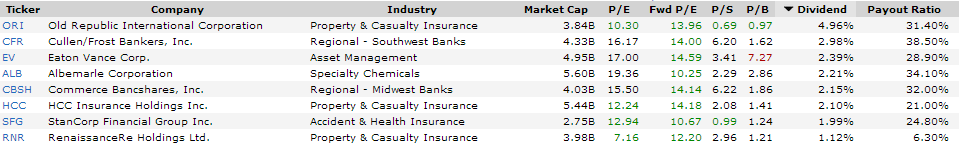# The Cheapest Dividend Growers From The MidCap 400 Dividend Aristocrats Index

I’ve recently looked at the S&P MidCap 400 Dividend Aristocrats Index in order to find new investment ideas.

These are my favorite stocks…

Albemarle (NYSE:ALB) has a market capitalization of \$5.63 billion. The company employs 4,231 people, generates revenue of \$2,445.55 million and has a net income of \$194.70 million.

Albemarle’s earnings before interest, taxes, depreciation and amortization (EBITDA) amounts to \$430.98 million. The EBITDA margin is 17.62 percent (the operating margin is 11.09 percent and the net profit margin 7.96 percent).

Financials: The total debt represents 56.18 percent of Albemarle’s assets and the total debt in relation to the equity amounts to 215.83 percent. Due to the financial situation, a return on equity of 13.58 percent was realized by Albemarle.

Twelve trailing months earnings per share reached a value of \$2.57. Last fiscal year, Albemarle paid \$0.82 in the form of dividends to shareholders.

Market Valuation: Here are the price ratios of the company: The P/E ratio is 19.36, the P/S ratio is 2.27 and the P/B ratio is finally 2.84. The dividend yield amounts to 2.23 percent and the beta ratio has a value of 1.68.Long-Term Stock Price Chart Of Albemarle (ALB)

Commerce Bancshares (NASDAQ:CBSH) has a market capitalization of \$4.04 billion. The company employs 4,728 people, generates revenue of \$648.29 million and has a net income of \$262.78 million.

Albemarle’s earnings before interest, taxes, depreciation and amortization (EBITDA) amounts to \$430.98 million. The EBITDA margin is 17.62 percent (the operating margin is 11.09 percent and the net profit margin 7.96 percent).

Financials: The total debt represents 8.20 percent of Commerce Bancshares’s assets and the total debt in relation to the equity amounts to 84.40 percent. Due to the financial situation, a return on equity of 11.72 percent was realized by Commerce Bancshares.

Twelve trailing months earnings per share reached a value of \$2.70. Last fiscal year, Commerce Bancshares paid \$0.86 in the form of dividends to shareholders.

Market Valuation: Here are the price ratios of the company: The P/E ratio is 15.49, the P/S ratio is 3.74 and the P/B ratio is finally 1.83. The dividend yield amounts to 2.17 percent and the beta ratio has a value of 1.01.Long-Term Stock Price Chart Of Commerce Bancshares (CBSH)Long-Term Dividend Payment History of Commerce Bancshares (CBSH)

Old Republic (NYSE:ORI) has a market capitalization of \$3.84 billion. The company employs 7,900 people, generates revenue of \$5,530.70 million and has a net income of \$409.90 million.

Old Republic’s earnings before interest, taxes, depreciation and amortization (EBITDA) amounts to \$635.20 million. The EBITDA margin is 11.48 percent (the operating margin is 11.48 percent and the net profit margin 7.41 percent).

Financials: The total debt represents 5.68 percent of Old Republic’s assets and the total debt in relation to the equity amounts to 24.59 percent. Due to the financial situation, a return on equity of 10.64 percent was realized by Old Republic.

Twelve trailing months earnings per share reached a value of \$1.43. Last fiscal year, Old Republic paid \$0.73 in the form of dividends to shareholders.

Market Valuation: Here are the price ratios of the company: The P/E ratio is 10.33, the P/S ratio is 0.69 and the P/B ratio is finally 0.96. The dividend yield amounts to 5.01 percent and the beta ratio has a value of 0.98.Long-Term Stock Price Chart Of Old Republic (ORI)8 Cheapest Stocks From The MidCap 400 Dividend Aristocrats Index (click to enlarge); Source: long-term-investments.blogspot.com